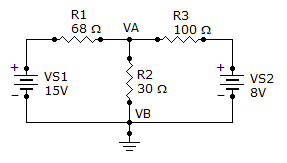# Electrical Engineering - Branch, Loop and Node Analyses - Discussion

### Discussion :: Branch, Loop and Node Analyses - General Questions (Q.No.4)

4.

Find the node voltage VA.[A]. 518 mV [B]. 5.18 V [C]. 9.56 V [D]. 956 mV

Explanation:

Reason: The voltage drop through R2 is 5.18v.

 Sundar said: (Apr 1, 2011) Open the following page: http://www.indiabix.com/electronics-circuits/ohms-law/ Start simulation. Right click on simulator -> File -> Import -> Paste the following code -> Click Import. \$ 1 5.0E-6 16.13108636308289 50 5.0 50 v 96 240 96 160 0 0 40.0 15.0 0.0 0.0 0.5 v 416 240 416 160 0 0 40.0 8.0 0.0 0.0 0.5 w 96 160 160 160 0 w 416 160 352 160 0 r 160 160 272 160 0 68.0 r 272 160 352 160 0 100.0 r 272 160 272 240 0 30.0 w 96 240 272 240 0 w 272 240 416 240 0 g 272 240 272 256 0 Then you will see the simulation.

 Selva said: (Mar 8, 2012) Using kirchoff's current law at node A, ((Va-15)/68)+((Va/30))+((Va-8)/100)=0 (75Va-1125+170Va+51Va-408/5100)=0 296Va=1533 Va=5.179=5.18 volts

 Shriki said: (Apr 3, 2016) Va/68-15/68 + Va/30 + Va/100-8/100 = 0. Va(1/68 + 1/30 + 1/100) - 15/68-8/100 = 0. 74Va/1275 = -511/1700. Va = (511*1275)/(1700*74). Va = 5.179V.

 Mani said: (Jun 18, 2016) Va (1/68 + 1/30 + 1/100) - 15/68 - 8/100 = 0. 74Va/1275 = - 511/1700. Va = (511 * 1275) / (1700 * 74). Va = 5.179V.

 Shareef said: (Dec 11, 2016) Any other way to solve this?

 Muhammad Farooq said: (May 13, 2019) Apply KCL at VA. Va/68-15/68 + Va/30 + Va/100-8/100 = 0. Va(1/68 + 1/30 + 1/100) - 15/68-8/100 = 0. 0.05804Va-0.3006 = 0, 0.05804Va = 0.3006, Va = 0.3006/0.05804. Va = 5.18.